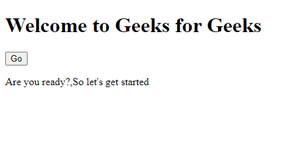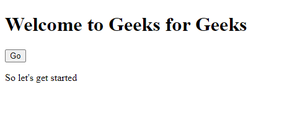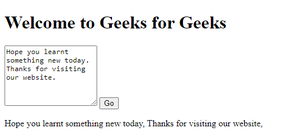Related Articles

# How to split multiline string into an array of lines in JavaScript ?

• Last Updated : 13 Apr, 2021

Multiline string in JavaScript means a string having two or more lines. To split a multiline string to an array we need to use split() in our JavaScript code.

split(separator, limit): The split() function is used to split the data depending upon the attributes we are passing in it. The separator attributes specify that from this word/sign the string will be divided. The limit attribute is optional, it specifies how many splits will be there.

Example 1:

## HTML

 ```<``html``>`` ` `<``body``>`` ` `    ``<``h1``>Welcome to Geeks for Geeks`` ` `    ``<``button` `onclick``=``"myFunction()"``>``        ``Go``    ```` ` `    ``<``p` `id``=``"StringToArray"``>`` ` `    ``<``script``>``        ``function myFunction() {``            ``var string = "Are you ready?" ``                ``+ "<``br``>So let's get started";`` ` `            ``var array = string.split("<``br``>");`` ` `            ``document.getElementById("StringToArray")``                ``.innerHTML = array;``        ``}``    `````` ` ``

Output:In this, when we will click on the “Go” button the array of the multiline string will be displayed on the screen separated by “,”.

Example 2: Now, let’s see how to get a particular index of an array.

## HTML

 ```<``html``>`` ` `<``body``>`` ` `    ``<``h1``>Welcome to Geeks for Geeks`` ` `    ``<``button` `onclick``=``"myFunction()"``>Go`` ` `    ``<``p` `id``=``"StringToArray"``>`` ` `    ``<``script``>``        ``function myFunction() {``            ``var string = "Are you ready?" ``                ``+ "<``br``>So let's get started";`` ` `            ``var array = string.split("<``br``>");`` ` `            ``document.getElementById("StringToArray")``                ``.innerHTML = array;``        ``}``    `````` ` ``

Output:As we wrote array, hence only the 2nd line has been printed. If we will write array, then it will be undefined as this array contains data in the first two indexes only that are 0 and 1 respectively.

Example 3: Now, let’s try to take string as an user input.

## HTML

 ```<``html``>`` ` `<``body``>``    ``<``h1``>Welcome to Geeks for Geeks`` ` `    ``<``textarea` `id``=``"write"` `        ``placeholder``=``"Write something"` `        ``style``=``"height:100px;"``>``    ```` ` `    ``<``button` `onclick``=``"myFunction()"``>Go`` ` `    ``<``p` `id``=``"StringToArray"``>`` ` ` ` `    ``<``script``>``        ``function myFunction() {`` ` `            ``var string = document``                ``.getElementById("write").value;`` ` `            ``var array = string.split(".");`` ` `            ``document.getElementById("StringToArray")``                ``.innerHTML = array;``        ``}``    `````` ` ``

Output:Hey geek! The constant emerging technologies in the world of web development always keeps the excitement for this subject through the roof. But before you tackle the big projects, we suggest you start by learning the basics. Kickstart your web development journey by learning JS concepts with our JavaScript Course. Now at it’s lowest price ever!

My Personal Notes arrow_drop_up